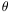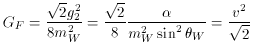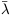### 3. FIXED AND TUNABLE PARAMETERS OF PHYSICS

3.1. The Standard Model and Everyday Life

The Standard Model of fundamental quantum fields has at least 20 adjustable parameters (including for this count Einstein's classical theory of gravity), although it explains almost all natural phenomena with less than half of these, and the basic structures are fixed by just a handful of them. At a deeper level, the values of the parameters are presumed to be not all truly independent and adjustable; symmetries fix relationships between some of them.

The minimal Standard Model has 19 ``adjustable'' parameters (Cahn 1996, Gaillard et al. 1999): Yukawa coefficients fixing the masses of the six quark and three lepton flavors (u, d, c, s, t, b, e, µ,), the Higgs mass and vacuum expectation value v (which multiplies the Yukawa coefficients to determine the fermion masses), three angles and one phase of the CKM (Cabibbo-Kobayashi-Maskawa) matrix (which mixes quark weak- and strong-interaction eigenstates), a phase for the quantum chromodynamic (QCD) vacuum, and three coupling constants g1, g2, g3 of the gauge group, U(1) x SU(2) x SU(3). If as seems likely the neutrinos are not massless, there are seven more parameters for them (three masses and another four CKM matrix elements).

Various more or less observable combinations of these parameters appear in discussing phenomenology, taking account of the change of couplings with energy. The traditional zero-energy electromagnetic fine structure constant= e2 = 1/137.03599, changes with energy scale to(mZ)1/128 at the Z mass scale; it is related to the electroweak parameters by e = g2 sinW, where the weak mixing angle tanWg' / g2 also fixes the W and Z mass ratio, sin2W = 1 - (mW2 / mZ2), and for consistently normalized currents one defines g1 = sqrt(5/3)g'. The Fermi constant of weak interactions can be written(1)

where v = 246 GeV is the expectation value of the Higgs field. The strong couplingSg32 can be defined at some energy scale, says(= mZ) = 0.12; or, an energy scaleQCD200 MeV can be defined where the coupling diverges. The masses of protons and other hadrons are thereby approximately fixed by the value ofS at any energy. (3)

The Standard Model plus classical gravity describes all known physical phenomenology within the present-day universe. Everyday matter (indeed nearly all of the ``baryonic'' matter of the universe aside from energetic particles) is almost entirely made of the lightest first generation fermions. (4) Since we may take the strong coupling to be fixed at the proton mass scale, and the fermion masses enter mostly through their ratio to the nucleon mass, the basic structures (almost) just depend on four parameters, which we may take to be the three light fermion masses me, mu, md and the elecromagnetic coupling constant, plus gravity. (5) Newton's constant of universal gravitation G specifies the coupling of all forms of energy to gravity (which is usually regarded as outside the ``Standard Model''). In the next section we review how the gravitational coupling of nucleons Gmproton2 defines the relationship between the structure of the astronomical scales of the universe and those of the microworld.

The electron mass and fine structure constant together determine the basic behavior of atomic matter and its interaction with radiation - in other words, all of chemistry and biology. They enter in familiar combinations such as the classical electron radius re =/me, the Thomson cross sectionT = (8/3)(/ me)2, the electron Compton wavelengthe = me-1, and the Bohr radius aBohr = (me)-1. The Rydberg energy me2 / 2 sets the scale of atomic binding; atomic fine structure (spin-orbit) splittings depends on higher powers of, and splittings of molecular modes, which include electronic, vibrational and rotational states, depend on powers of me / mproton.

The detailed relationships among atomic and molecular eigenstates are not preserved continuously or homologously asand me are adjusted, and would be scrambled with even small changes. However, structural chemistry would not change much ifand me were adjusted slightly differently. The structure of electron orbitals in atoms and molecules scales homologously in first order with the Bohr radius, and the energy levels of the ground-state orbitals scale with the Rydberg. So, while it does seem miraculous that complementary structures can form with the specificity (say) of purines and pyramidines in DNA, the possibility of this miracle can be traced back to group theory and quantum mechnanics; ifand/or me changed, the DNA structure would remain almost the same, it would just change size relative to, say, the classical electron radius. (The departure from homology enters only in subdominant terms in the Hamiltonian, such as the spin-orbit or nucleus-nucleus interactions.)

This amazing achievement of quantum theory illuminates another good example of failed anthropic reasoning. Before quantum mechanics, it was suggested that atomic properties must have been tuned to achieve the marvellous chemical structures needed for life (Henderson 1913). Instead it appears that ordinary Darwinian natural selection has found and exploited the structural opportunities presented by underlying symmetries. Biology and not physics or cosmology should be given credit for this miracle!

By contrast, changing the quark masses even a small amount has drastic consequences which no amount of Darwinian selection can compensate. The u-d mass difference in particular attracts attention because the d is just enough heavier than u to overcome the electromagnetic energy difference to make the proton (uud) lighter than the neutron (udd) and therefore stable. On the other hand if it were a little heavier still, the deuteron would be unstable and it would be difficult to assemble any nuclei heavier than hydrogen. This then is a good candidate for selective tuning among multiverses. Similarly, the sum of the quark masses controls the pion mass, so changing them alters the range of the nuclear potential and significantly changes nuclear structure and energy levels. Even a small change radically alters the history of nuclear astrophysics, for example, by eliminating critical resonances of nucleosynthesis needed to produce abundant carbon (Hoyle et al. 1953). It would be surprising if symmetries conspired to satisfy these constraints, but quite natural if the parameters can adopt a continuous range of values. One therefore expects these particular parameters to continue to elude relationships fixed by symmetries.

3 The relation ofQCD toS(E) also depends on the fermion and Higgs masses, through threshold effects. Back.
4 The higher generations are less prominent in nature than the first because they are heavier and decay by weak interactions, although they are always present at some level because of mixing and probably play important roles in supernova physics and other exotic but important astrophysical environments such as neutron stars. They also enter through the CKM matrix, one complex phase of which is a source of observed CP violation and therefore possibly related to the physics responsible for creating the cosmic excess of matter over antimatter. The masses of the heavy fermions matter little to familiar natural phenomenology, so they could be set by the choices selectively adopted by the first generation if the fermion masses of the three generations are (as is conjectured) coupled to each other in a unified scheme by a mixing matrix. There are many such schemes proposed (Berezhiani 1996); for example, in the ``democratic'' scenario of Fukugita, Tanimoto and Yanagida (1999), the nine fermion masses are determined by five parameters, and still only two independent parameters determine the masses of u, d, e (with md / me fixed by SO(10)). Back.
5 Agrawal et al. (1998) have developed the point of view that the weak scale itself is determined anthropically and that v is the one tunable parameter - singled out in the standard model by having a dimension. Indeed the fundamental degrees of freedom of the fundamental theory are not known and one of the main objectives of studies such as these is to sniff them out. Here I imagine adjusting some coefficients in the Lagrangian according to the constraints imposed by unification. This amounts to exploring a different space of variation, with more degrees of freedom, than Agrawal et al. For most of the arguments presented here, it does not matter whether the Higgs is counted as a separate degree of freedom. Note however that tuning only the Higgs varies all the fermion masses in lockstep, and cannot by itself tune more than one degree of freedom. Back.